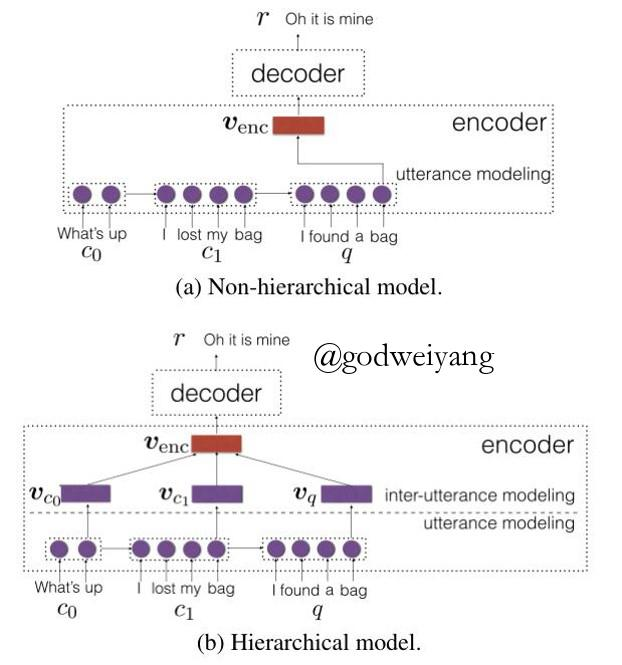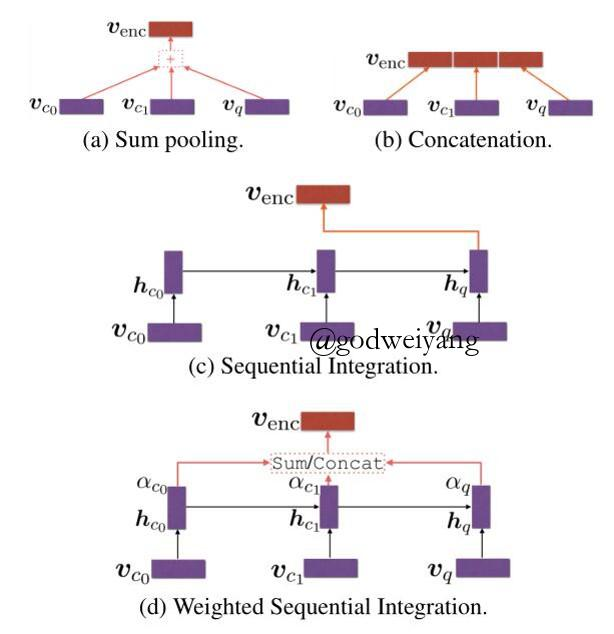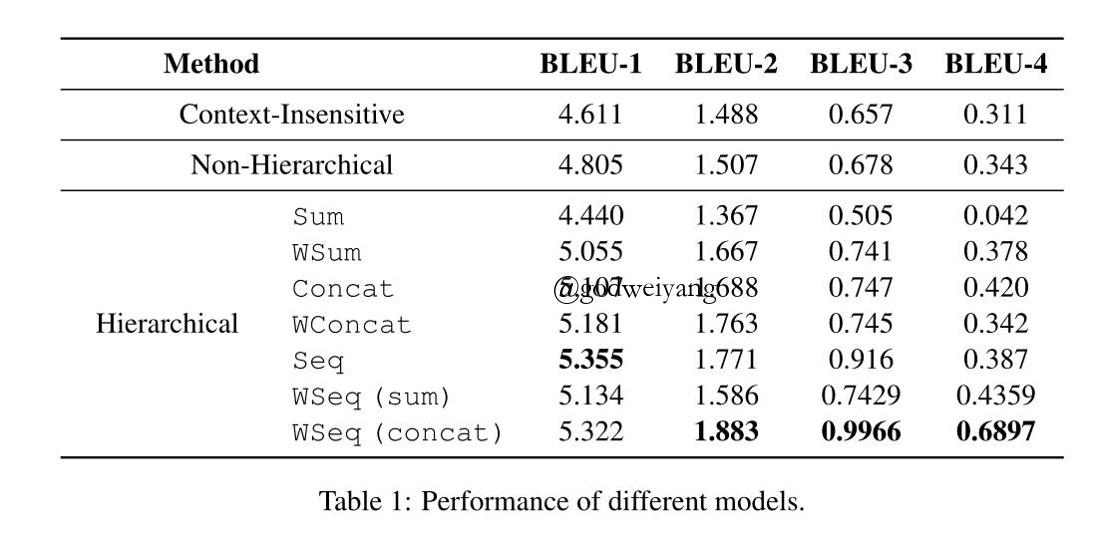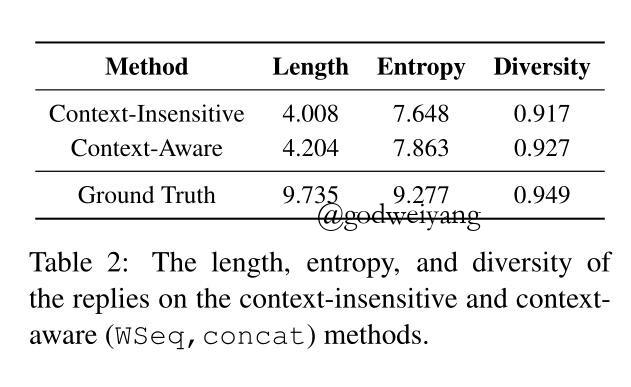How to Make Context More Useful? An Empirical Study on Context-Aware Neural Conversational Models# 模型

• 我们怎么样才能更好的利用上下文？
• 上下文对神经对话系统的影响是什么？${s_{ {c_i}}} = sim({c_i},q) = \frac{ { {e_{c_i} } \cdot {e_q}}}{ {\left\| {e_{c_i}} \right\| \cdot \left\| { {e_q}} \right\|}}$

$\alpha _{c_i} = \frac{ {\exp ({s_{ {c_i}}})}} { {\sum\nolimits_{j = 0}^n {\exp ({s_{ {c_i}}})} + \exp ({s_q})}}$
${ {\alpha _q} = \frac{ {\exp ({s_q})}}{ {\sum\nolimits_{j = 0}^n {\exp ({s_{ {c_i}}})} + \exp ({s_q})}}}$

• WSeq(sum):
${v_{enc}} = \sum\limits_{i = 0}^n { {\alpha _{ {c_i}}}{h_{ {c_i}}} + {\alpha _q}{h_q}}$
• WSeq(concat):
${v_{enc}} = \left[ { {\alpha _{ {c_0}}}{h_{ {c_0}}}; \ldots ;{\alpha _{ {c_n}}}{h_{ {c_n}}};{\alpha _q}{h_q}} \right]$

# 实验结果# 实验结果分析

• 我们怎么样才能更好的利用上下文？
首先我们发现采用上下文实验结果的确比不采用的更好了。然后分层的模型结果比不分层的更好。我们猜测原因可能是对话系统不同于其他NLP任务，对话系统句子可能出自不同的人。让每个上下文保持独立很重要，而不是简单的池化结合到一起，所以直接连接起来效果更好。上下文和查询相关性对系统有帮助。
• 上下文对神经对话系统的影响是什么？可以看出，采用上下文的模型能产生更长、更有意义、更多样性的回答。我们还发现了一个有趣的现象：一个encode-decoder模型如果想要生成有意义的回答，必须要足够多的有意义的信息提供给它。这解释了为什么seq2seq在其他NLP任务表现得很好，但是在对话系统表现得不好。

转载规则

《How to Make Context More Useful? An Empirical Study on Context-Aware Neural Conversational Models》 韦阳 采用 知识共享署名 4.0 国际许可协议 进行许可。
上一篇吴恩达深度学习公开课第一周学习笔记

2017-09-19保研第一，我还是选择留在了本校

2017-09-19
目录